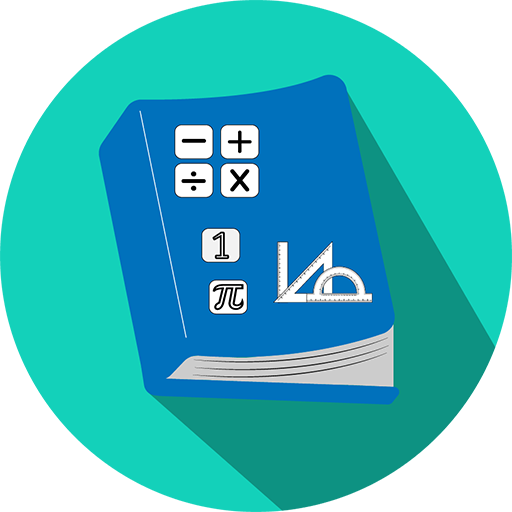# All Math Formula

### App Description

The following description comes from the Google Play Store description of the app:

All essential Math Formula for all android users. Offline content. You don't need internet to read formulas. In this app, you will get 1000+ math formulas and equations. With formula, you will get proper diagram, so that you will understand formula easily. You can read various equations easily from this app.

In this app, you will get mathematics formula and equations includes:

Algebra
Geometry
Trigonometry

Calculus:
Limits
Derivatives
Integrals

Basic Properties & Facts

Arithmetic Operations
Exponent Properties
Properties of Inequalities
Properties of Absolute Value
Distance Formula
Complex Numbers
Logarithms and Log Properties
Factoring and Solving

Factoring Formulas
Square Root Property
Absolute Value Equations/Inequalities
Completing the Square
Functions and Graphs

Constant Function
Line/Linear Function
Circle
Ellipse
Hyperbola

Square
Rectangle
Circle
Triangles
Parallelogram
Trapezoid
Cube
Cylinder
Sphere
Cone
All in one
Geometric Symbols

Trigonometry Functions
Special Angles
Trigonometric Function Values in Quadrants II, III, and IV
The Unit Circle
Double Angle Formulas
Half Angle Formulas
Power Reducing Formulas
Product‐to‐Sum Formulas
Cofunctions Formulas
Law of Sines
Law of Cosines
Law of Tangents
Pythagorean Identities (for any angle θ)
Mollweide’s Formula

Limits Definitions
Relationship between the limit and one-sided limits
Limits Properties Formulas
Basic Limit Evaluations Formulas
Evaluation Techniques Formulas
Some Continuous Functions
Intermediate Value Theorem

Derivatives Definition and Notation
Interpretation of the Derivative
Basic Properties and Formulas
Common Derivatives
Chain Rule Variants
Higher Order Derivatives
Implicit Differentiation
Increasing/Decreasing – Concave Up/Concave Down
Extrema
Mean Value Theorem
Newton’s Method
Related Rates
Optimization

Integrals Definitions
Fundamental Theorem of Calculus
Properties
Common Integrals
Standard Integration Techniques
Improper Integral
Approximating Definite Integrals
Sensitive Permissions Used By This App
Permission What Why
Full network access Can access the internet It appears this app uses this data for internal use within the app's functionality

### Privacy Analysis

Version of the app analyzed:

1.7

App was last analyzed by Privacy Grade on:

02/25/2015

Why does this app have this grade?

Our method for grading apps uses a privacy model that we built. This model is based on crowdsourced surveys that we conducted to capture people’s expectations and comfort levels with various app behaviors.

See the sensitive permissions table below the app description to see the permissions that made users of the app uncomfortable.

If you have issues about the information on this page, click the button below to send us feedback.